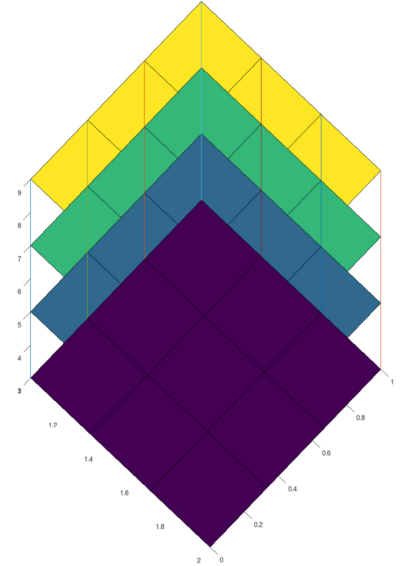UNB/ CS/ David Bremner/ teaching/ cs2613/ labs/ Lab 21

Time
10 minutes
Activity
Group discussion

# K nearest neighbour

Time
25 minutes
Activity
Individual

The `k`-Nearest neighbour algorithm (KNN) is a famous machine learning or pattern classification algorithm. In this algorithm you are given a training set of numerical measurement vectors (e.g. lab attendance and height) each labelled with a class (e.g. final grade). Given a new, unlabelled measurement, the algorithm predicts the corresponding class by looking at the `k`-closest training vectors, and voting.

In this lab, and in A6, we will work with a famous set of measurements of iris flowers), where the "class" is the species of flower.

You need to know the following definition from linear algebra

``````distance(X,Y) = norm(X-Y)
``````

Complete the following function which returns the row indices of the `k` closest rows of `data` to `v`. In our data sets, we will assume the first column is always the class label, and should ignored in distance calculations.

```function out = nearest(v, k, data)
for i=1:rows(data)
dist(i)=
endfor
[sorted, indices]=sort(dist);
out = sort(indices(1:k));
endfunction

%!test
%! v = [0,1,1]
%! data = [0,0,0; 0,0,1; 0,1,0; 1,1,1]
%! assert(nearest(v,1,data) == 4)
%! assert(nearest(v,3,data) == [2,3,4])
```

You can time your nearest neighbour query with the following:

• save training.mat as `~/fcshome/cs2613/labs/L21/training.mat`
• save testing.mat as `~/fcshome/cs2613/labs/L21/testing.mat`
• Create a file `~/fcshome/cs2613/labs/L21/bench.m` with the following content (timeit can be downloaded)
```load training
timeit(1000,@nearest,testing(1,:), 3, training);
```

# Visualizing boxes

Time
15 minutes
Activity
Individual / Demo

In Assignment 6 voting is done in a different way. Rather than voting amoung the `k` closest points to a query vector, the vectors from each class in a given "bucket" or box region of feature space are counted.

In the following example, the columns of the array `ranges` define the intervals of values for each feature, and `p` defines the "precision", or how many sub-intervals each feature is divided into. In this part we stick to 3 features because that is all we can easily visualize. Fill in values for `p` and `ranges` so that the plot looks something like```ranges = [ , , ;
, , ]
p=

x = linspace(ranges(1,1),ranges(2,1),p+1)
y = linspace(ranges(1,2),ranges(2,2),p+1)
z = linspace(ranges(1,3),ranges(2,3),p+1)

[X,Y] = meshgrid(x,y)

clf
axis([ranges(1,1),ranges(2,1),ranges(1,2),ranges(2,2),ranges(1,3),ranges(2,3)],"equal")
hold on
for i=1:columns(z)
surf(X,Y,ones(size(X))*z(i))
endfor
for i=1:columns(x)
for j=1:columns(y)
plot3(ones(size(z))*x(i),ones(size(z))*y(j),z)
endfor
endfor
```

# K nearest neighbours continued

Time
10 minutes
Activity
Demo

In practice the function `nearest` would probably be combined with something like the following; they are split out here to help with debugging and testing. Use the parameter `fun` here rather than calling `nearest` directly, so we can swap in a different query function later.

```function out=findclass(v, k, data, fun=@nearest)
selection=
out = mode(data(selection,1));
endfunction

%!test
%! v = [0,1,1];
%! data = [0,0,0; 0,0,1; 0,1,0; 1,1,1];
%! assert(findclass(v,1,data) == 1)
%! assert(findclass(v,3,data) == 0)
```

Here is a for-loop based version of a kNN classifier. The return value is the success rate of the classifier (i.e. the fraction of the time it gets the right answer on the test set).

```function ratio=knn(k,training, testing, output=0)

correct = 0;
for i=1:rows(testing)
row=testing(i,:);
if findclass(row,k,training) == row(1);
correct += 1;
endif
endfor

ratio = correct/rows(testing);
endfunction
```

You can try out the classifier with

```load testing
knn(3,testing,training)
```

# Vectorizing `nearest`

Time
20 minutes
Activity
individual

We need a couple of ideas from basic linear algebra to make this vectorization work. We can make many copies of the input vector `v` by multiplying it by a ones column vector on the left (no loop required). Try this in octave

``````ones(10,1)*[1,2,3,4]
``````

As a convenience, we can also sort by the square of the norm, which is simply the dot product of a vector with itself.

```function out = nearest2(v, k, data)

diff = data(       ) - ones(          ,1)*v(2:end);
normsq = dot(            )
[sorted, indices]=sort(normsq);
out = sort(transpose(indices(1:k)));
endfunction

%!test
%! v = [0,1,1];
%! data = [0,0,0; 0,0,1; 0,1,0; 1,1,1];
%! assert(nearest2(v,1,data) == 4)
%! assert(nearest2(v,3,data) == [2,3,4])
```

Finally, check the speedup with this second knn frunction

```function ratio=knn2(k,training, testing, output=0)

correct = 0;
for i=1:rows(testing)
row=testing(i,:);
if findclass(row,k,training,@nearest2) == row(1);
correct += 1;
endif
endfor

ratio = correct/rows(testing);
endfunction
```

You can use the follow lines in `bench.m`

```timeit(10,@knn,3,training,testing);
timeit(10,@knn2,3,training,testing);
```

# Using rowfun

Time
20 minutes
Activity
individual

Use the `find` function (along with rowfun from last lab) to complete the following loop-free version of `knn`. It turns out there's not a huge performance benefit, but the technique of using `find` is useful to know in general.

```function ratio=rowknn(k,training, testing)
classes=rowfun(@(row)(findclass(row,k,training)),testing);
correct=length(                           );
ratio = correct/rows(testing);
endfunction
```

Add the following line to your `bench.m`, and compare the timings.

```timeit(10,@rowknn,3,training,testing);
```

If you have time, it's interesting to look at the profile output for the 3 `knn` versions